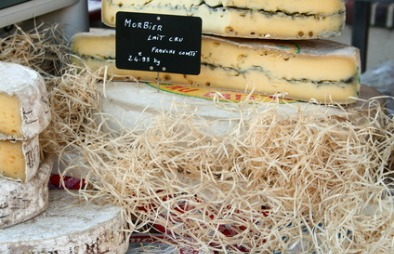# Metric ConverterConverts for weight, distance, volume, area, temperature

Metric to Imperial Conversion Table

Instructions:
Type a value into the lefthand column. Then
click in the righthand column to see the result.

Metric/Imperial
Converter
Go
To:
Length | Area | Mass
and Weight
| Liquid Volume | Temperature

Length

Type in what you know

Conversion Factor

Result

inches

x 25.4 =

millimetres

feet

x 30.48 =

centimetres

yards

x 0.9144 =

metres

miles

x 1.609 =

kilometres

millimetres

x 0.03937 =

inches

centimetres

x 0.3937 =

inches

metres

x 1.0936 =

yards

kilometres

x 0.6215 =

miles

Go
To:
Length | Area | Mass
and Weight
| Liquid Volume | Temperature

Area

Type in what you know

Conversion Factor

Result

sq. inches

x 6.452 =

sq cm

sq. feet

x 0.09 =

sq metres

sq. yards

x 0.8361 =

sq metres

sq. miles

x 2.590 =

sq kilometres

sq. acres

x 0.4 =

hectares

sq. cm

x 0.155 =

sq inches

sq. metres

x 1.196 =

sq yards

sq. km

x 0.3861 =

sq miles

sq. hectares

x 2.5 =

acres

Go
To:
Length | Area | Mass
and Weight
| Liquid Volume | Temperature

Mass and Weight

Type in what you know

Conversion Factor

Result

ounces

0.035=

grams

pounds

x 0.4536 =

kilograms

US tons

x 0.9 =

metric tons

grams

x28.35=

ounces

kilograms

x 2.2046 =

pounds

metric tons

x 1.1 =

US tons

Go
To:
Length | Area | Mass
and Weight
| Liquid Volume | Temperature

Liquid Volume

Type in what you know

Conversion Factor

Result

fluid ounces

x 30 =

millilitres

pints

x 0.568 =

litres

quarts

x 0.95 =

litres

gallons (UK)

x 4.54 =

litres

millilitres

x 0.034 =

fluid ounces

litres

x 2.1 =

pints

litres

x 1.06 =

quarts

litres

x 0.22 =

gallons (UK)

Go
To:
Length | Area | Mass
and Weight
| Liquid Volume | Temperature

Temperature

Type in what you know

Conversion Factor

Result

Fahrenheit

– 32 x 5/9 =

Celsius

Celsius

x 9/5 + 32=

Fahrenheit